# Calculating Probability

## Learn About Calculating Probability With The Following Examples And Interactive Exercises.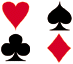Problem: A spinner has 4 equal sectors colored yellow, blue, green and red. What are the chances of landing on blue after spinning the spinner? What are the chances of landing on red?Solution: The chances of landing on blue are 1 in 4, or one fourth.

The chances of landing on red are 1 in 4, or one fourth.

This problem asked us to find some probabilities involving a spinner. Let's look at some definitions and examples from the problem above.

 Definition Example An experiment is a situation involving chance or probability that leads to results called outcomes. In the problem above, the experiment is spinning the spinner. An outcome is the result of a single trial of an experiment. The possible outcomes are landing on yellow, blue, green or red. An event is one or more outcomes of an experiment. One event of this experiment is landing on blue. Probability is the measure of how likely an event is. The probability of landing on blue is one fourth.

In order to measure probabilities, mathematicians have devised the following formula for finding the probability of an event.

Probability Of An Event
 P(A) = The Number Of Ways Event A Can Occur The total number Of Possible Outcomes

The probability of event A is the number of ways event A can occur divided by the total number of possible outcomes. Let's take a look at a slight modification of the problem from the top of the page.

Experiment 1: A spinner has 4 equal sectors colored yellow, blue, green and red. After spinning the spinner, what is the probability of landing on each color?

The possible outcomes of this experiment are yellow, blue, green, and red.

Probabilities:

 P(yellow) = # of ways to land on yellow = 1 total # of colors 4 P(blue) = # of ways to land on blue = 1 total # of colors 4 P(green) = # of ways to land on green = 1 total # of colors 4 P(red) = # of ways to land on red = 1 total # of colors 4

Experiment 2: A single 6-sided die is rolled. What is the probability of each outcome? What is the probability of rolling an even number? of rolling an odd number?Outcomes: The possible outcomes of this experiment are 1, 2, 3, 4, 5 and 6.

Probabilities:

 P(1) = # of ways to roll a 1 = 1 total # of sides 6 P(2) = # of ways to roll a 2 = 1 total # of sides 6 P(3) = # of ways to roll a 3 = 1 total # of sides 6 P(4) = # of ways to roll a 4 = 1 total # of sides 6 P(5) = # of ways to roll a 5 = 1 total # of sides 6 P(6) = # of ways to roll a 6 = 1 total # of sides 6 P(even) = # ways to roll an even number = 3 = 1 total # of sides 6 2 P(odd) = # ways to roll an odd number = 3 = 1 total # of sides 6 2

Experiment 2 illustrates the difference between an outcome and an event. A single outcome of this experiment is rolling a 1, or rolling a 2, or rolling a 3, etc. Rolling an even number (2, 4 or 6) is an event, and rolling an odd number (1, 3 or 5) is also an event.

In Experiment 1 the probability of each outcome is always the same. The probability of landing on each color of the spinner is always one fourth. In Experiment 2, the probability of rolling each number on the die is always one sixth. In both of these experiments, the outcomes are equally likely to occur. Let's look at an experiment in which the outcomes are not equally likely.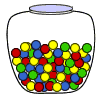Experiment 3: A glass jar contains 6 red, 5 green, 8 blue and 3 yellow marbles. If a single marble is chosen at random from the jar, what is the probability of choosing a red marble? a green marble? a blue marble? a yellow marble?

Outcomes: The possible outcomes of this experiment are red, green, blue and yellow.

Probabilities:

 P(red) = # of ways to choose red = 6 = 3 total # of marbles 22 11 P(green) = # of ways to choose green = 5 total # of marbles 22 P(blue) = # of ways to choose blue = 8 = 4 total # of marbles 22 11 P(yellow) = # of ways to choose yellow = 3 total # of marbles 22

The outcomes in this experiment are not equally likely to occur. You are more likely to choose a blue marble than any other color. You are least likely to choose a yellow marble.

Experiment 4: Choose a number at random from 1 to 5. What is the probability of each outcome? What is the probability that the number chosen is even? What is the probability that the number chosen is odd?

Outcomes: The possible outcomes of this experiment are 1, 2, 3, 4 and 5.

Probabilities:

 P(1) = # of ways to choose a 1 = 1 total # of numbers 5 P(2) = # of ways to choose a 2 = 1 total # of numbers 5 P(3) = # of ways to choose a 3 = 1 total # of numbers 5 P(4) = # of ways to choose a 4 = 1 total # of numbers 5 P(5) = # of ways to choose a 5 = 1 total # of numbers 5 P(even) = # of ways to choose an even number = 2 total # of numbers 5 P(odd) = # of ways to choose an odd number = 3 total # of numbers 5

The outcomes 1, 2, 3, 4 and 5 are equally likely to occur as a result of this experiment. However, the events even and odd are not equally likely to occur, since there are 3 odd numbers and only 2 even numbers from 1 to 5.

Summary: The probability of an event is the measure of the chance that the event will occur as a result of an experiment. The probability of an event A is the number of ways event A can occur divided by the total number of possible outcomes. The probability of an event A, symbolized by P(A), is a number between 0 and 1, inclusive, that measures the likelihood of an event in the following way:

• If P(A) > P(B) then event A is more likely to occur than event B.
• If P(A) = P(B) then events A and B are equally likely to occur.

### Exercises

Directions: Read each question below. Select your answer by clicking on its button. Feedback to your answer is provided in the RESULTS BOX. If you make a mistake, choose a different button.

 1. Which of the following is an experiment? Tossing a coin.Rolling a single 6-sided die.Choosing a marble from a jar.All of the above. RESULTS BOX:
 2. Which of the following is an outcome? Rolling a pair of dice.Landing on red.Choosing 2 marbles from a jar.None of the above. RESULTS BOX:
 3. Which of the following experiments does NOT have equally likely outcomes? Choose a number at random from 1 to 7.Toss a coin.Choose a letter at random from the word SCHOOL.None of the above. RESULTS BOX:
 4. What is the probability of choosing a vowel from the alphabet?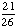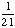None of the above. RESULTS BOX:
 5. A number from 1 to 11 is chosen at random. What is the probability of choosing an odd number?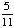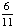None of the above. RESULTS BOX: